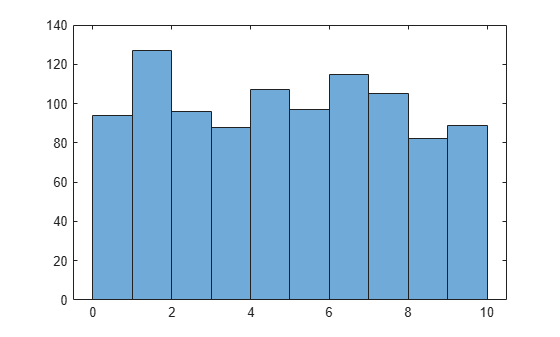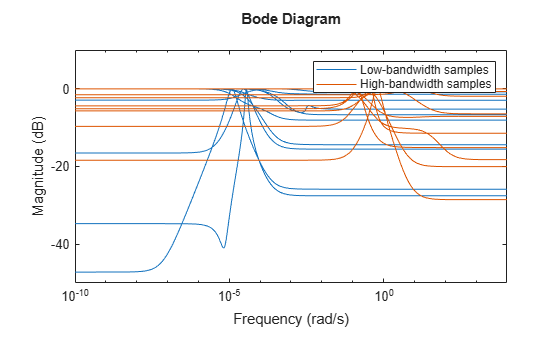usample

Generate random samples of uncertain or generalized model

Syntax

B = usample(A);
B = usample(A,N)
[B,SampleValues] = usample(A,N)
[B,SampleValues] = usample(A,Names,N)
[B,SampleValues] = usample(A,Names1,N1,Names2,N2,...)
[B,SampleValues] = usample(A,N,Wmax)
[B,SampleValues] = usample(A,Names,N,Wmax)

Description

B = usample(A) substitutes a random sample of the uncertain objects in A, returning a certain (i.e., not uncertain) array of size [size(A)]. The input A can be any uncertain element, matrix, or system, such as ureal, umat, uss, or ufrd. A can also be any generalized matrix or system, such as genss or genmat, that contains uncertain blocks and other types of Control Design Blocks. If A contains non-uncertain control design blocks, these are unchanged in B. Thus, for example, usample applied to a genss with both tunable and uncertain blocks, the result is a genss array with only tunable blocks.

B = usample(A,N) substitutes N random samples of the uncertain objects in A, returning a certain (i.e., not uncertain) array of size [size(A) N].

[B,SampleValues] = usample(A,N) additionally returns the specific sampled values (as a Struct whose field names are the names of A's uncertain elements) of the uncertain elements. Hence, B is the same as usubs(A,SampleValues).

[B,SampleValues] = usample(A,Names,N) samples only the uncertain elements listed in the Names variable (cell, or char array). If Names does not include all the uncertain objects in A, then B will be an uncertain object. Any entries of Names that are not elements of A are simply ignored. Note that usample(A,fieldnames(A.Uncertainty),N) is the same as usample(A,N).

[B,SampleValues] = usample(A,Names1,N1,Names2,N2,...) takes N1 samples of the uncertain elements listed in Names1, and N2 samples of the uncertain elements listed in Names2, and so on. size(B) will equal [size(A) N1 N2 ...].

The scalar parameter Wmax in

[B,SampleValues] = usample(A,N,Wmax)
[B,SampleValues] = usample(A,Names,N,Wmax)
[B,SampleValues] = usample(A,Names,N,Wmax)

affects how ultidyn and umargin elements within A are sampled, restricting the poles of the samples. If A is a continuous-time uss or ufrd, then the poles of sampled GainBounded ultidyn or umargin elements in SampleValues will each have magnitude <= BW. If A is a discrete-time, then sampled GainBounded ultidyn or umargin elements are obtained by Tustin transformation, using BW/(2*TS) as the (continuous) pole magnitude bound. In this case, BW should be < 1. If the ultidyn type is PositiveReal, then the samples are obtained by bilinearly transforming (see Normalizing Functions for Uncertain Elements) the GainBounded elements described above.

Examples

collapse all

Create a real uncertain parameter, sample it, and plot a histogram of the sampled values.

A = ureal('A',5);
Asample = usample(A,500);

Examine the size of the parameter and the sample array.

size(A)
Uncertain real scalar.
size(Asample)
ans = 1×3

1     1   500

A is a scalar parameter. The dimensions of Asample reflect that A is a 1-by-1 parameter. Examine the data type of Asample.

class(Asample)
ans =
'double'

The samples of the scalar parameter are numerical values.

Plot the histogram of sampled values.

hist(Asample(:))This example illustrates how to sample the open and closed-loop response of an uncertain plant model for Monte Carlo analysis.

Create two uncertain real parameters and an uncertain plant.

gamma = ureal('gamma',4);
tau = ureal('tau',.5,'Percentage',30);
P = tf(gamma,[tau 1]);

Create an integral controller based on the nominal values of plant uncertainties.

KI = 1/(2*tau.Nominal*gamma.Nominal);
C = tf(KI,[1 0]);

Now create an uncertain closed-loop system.

CLP = feedback(P*C,1);

Sample the plant at 20 values, distributed uniformly about the tau and gamma parameter cube.

[Psample1D,Values1D] = usample(P,20);
size(Psample1D)
20x1 array of state-space models.
Each model has 1 outputs, 1 inputs, and 1 states.

This sampling returns an array of 20 state-space models, each representing the closed-loop system within the uncertainty.

Now sample the plant at 10 values of tau and 15 values of gamma.

[Psample2D,Values2D] = usample(P,'tau',10,'gamma',15);
size(Psample2D)
10x15 array of state-space models.
Each model has 1 outputs, 1 inputs, and 1 states.

Plot the step responses of the 1-D sampled plant.

subplot(2,1,1);
step(Psample1D)Evaluate the uncertain closed-loop model at the same values using usubs, and plot the step response.

subplot(2,1,2);
step(usubs(CLP,Values1D))To see the effect of limiting the bandwidth of sampled models with Wmax, create two ultidyn objects.

A = ultidyn('A',[1 1]);
B = ultidyn('B',[1 1]);

Sample 10 instances of each, using a bandwidth limit of 1 rad/sec on A, and 20 rad/sec on B.

Npts = 10;
As = usample(A,Npts,1);
Bs = usample(B,Npts,20);

Plot 10-second step responses, for the two sample sets.

step(As,'r',Bs,'b--',10)The lower bandwidth limit on the samples of A results in generally slower step responses for those samples.

Compatibility Considerations

expand all

Behavior changed in R2020a